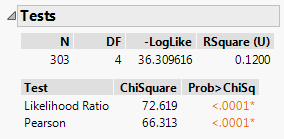Publication date: 11/10/2021

# Tests

The Tests report shows the results for two tests to determine whether the response level rates are the same across X levels.

To produce the report shown in Figure 7.8, follow the instructions in Example of Contingency Analysis.

Figure 7.8 Example of a Tests ReportNote the following about the Chi-square statistics:

When both categorical variables are responses (Y variables), the Chi-square statistics test that they are independent.

You might have a Y variable with a fixed X variable. In this case, the Chi-square statistics test that the distribution of the Y variable is the same across each X level.